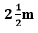## Ex 5.4 Question 1.

Which term of the AP: 121, 117, 113, . . ., is its first negative term? [Hint: Find n for an < 0]

Solution:

a = 121

d = 117-121= -4

an = a+(n −1)d

an = 121+(n−1)(-4)

= 121-4n+4

=125-4n

To find the first negative term of A.P.

Therefore, a< 0

125-4n < 0

125 < 4n

n>125/4

n>31.25

Therefore, 32nd term will be the first negative term of this A.P.

## Ex 5.4 Question 2.

The sum of the third and the seventh terms of an AP is 6 and their product is 8. Find the sum of first sixteen terms of the AP.

Solution:

Let the first term = a

Common difference = d

The sum of the third and the seventh terms of an AP is 6

a3 + a7 = 6

⇒ a + 2d + a +6d = 6

⇒ 2a + 8d = 6

⇒ a + 4d = 3

⇒ a = 3 – 4d ……(i)

The product of the third and the seventh terms of an AP is 8

a×a= 8

⇒ (a +2d)(a +6d) = 8

Putting the value of a from equation (i)

(3 – 2d) (3 + 2d) = 8

⇒ 3² – 4d² = 8

⇒ 4d² = 1

⇒ d = ±½

If d = ½

Putting the value of d in equation (i)

a = 3 – 4(½) = 1

The sum of 16 terms of A.P.

Sn = n/2 [2a+(n-1)d]

S16 = 16/2 [2 +(16-1)1/2] = 8(2+15/2) = 76

d= -1/2

Putting the value of d in euqation (i)

a = 3 – 4 (-1/2) = 5

Then, the sum of the16 terms of AP.

S16 = 16/2 [2.5+(16-1)(-1/2)] = 8(5/2)=20

## Ex 5.4 Question 3.

A ladder has rungs 25 cm apart. (see Fig. 5.7). The rungs decrease uniformly in length from 45 cm at the bottom to 25 cm at the top. If the top and the bottom rungs areapart, what is the length of the wood required for the rungs? [Hint: Number of rungs = -250/25 ].Solution:

The distance between top and bottom rungs is 2½ m and the distance between two successive rungs is 25 cm.

∴The number of rungs = 250/25 + 1 = 11

The length of rungs is increasing from 25cm to 45cm in the form of A.P. Whose,

a = 45

l = 25

n = 11

Sn= n/2(a+ l)

Sn= 11/2(45+25) = 11/2(70) = 385 cm

Hence, 385cm length of the wood required for the rungs.

## Ex 5.4 Question 4.

The houses of a row are numbered consecutively from 1 to 49. Show that there is a value of x such that the sum of the numbers of the houses preceding the house numbered x is equal to the sum of the numbers of the houses following it. Find this value of x. [Hint :Sn-1 = S49 – Sx ]

Solution:

a = 1

d=1

Sum of n term of an A.P.

Sn = n/2 [2a+(n-1)d]

= (x-1)/2[2.1+(x-1-1)1]

= (x-1)/2 [2+x-2]

= x(x-1)/2 …………(i)

S49 – Sx = {49/2[2.1+(49-1)1]}–{x/2[2.1+(x-1)1]}

= 25(49) – x(x + 1)/2 ………….(ii)

According to the given condition, equation(i) and equation(ii) are equal to each other;

Therefore,

x(x-1)/2 = 25(49) – x(x-1)/2

x = ±35

Hence, the value of x is 35.

## Ex 5.4 Question 5.

A small terrace at a football ground comprises of 15 steps each of which is 50 m long and built of solid concrete. Each step has a rise of 1 4 m and a tread of 1 2 m. (see Fig. 5.8). Calculate the total volume of concrete required to build the terrace. [Hint : Volume of concrete required to build the first step = ¼ ×1/2 ×50 m3.]Solution:

The volume of concrete required to build the first step = ¼ ×½ ×50 = ¼ x 25 m³

The volume of concrete required to build the second step =2/4 ×½/×50 = 2/4 x 25 m³

The volume of concrete required to build the third step = ¾ ×½ ×50 =¾ x 25 m³

a = 25/4

d = 2/4 x 25 – ¼ x 25 = ¼ x 25

Sn = n/2[2a+(n-1)d]

= 15/2[2×(¼ x 25 )+(15 -1)¼ x 25]

= 15/2 [25/2+175/2]

= 15/2 x 200/2

Sn = 750m³

Hence, the total 750m³ volume of concrete required to build the terrace.

error: Content is protected !!